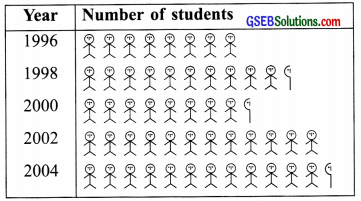# GSEB Solutions Class 6 Maths Chapter 9 Data Handling Ex 9.2

Gujarat Board GSEB Textbook Solutions Class 6 Maths Chapter 9 Data Handling Ex 9.2 Textbook Questions and Answers.

## Gujarat Board Textbook Solutions Class 6 Maths Chapter 9 Data Handling Ex 9.2

Question 1.
The total number of animals in the five villages are as follows:
Village A: 80
Village B: 120
Village C: 90
Village D: 40
Village E: 60
Prepare a pictograph of these animals using one symbol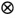to represent 10 animals and answer the following questions:
(a) How may symbols represent animals of village E?
(b) Which village has the maximum number of animals?
(c) Which village has more animals: village A or village C?
Solution:
We have the following pictograph using the given data, such that.represents 10 animals.(a) 6 symbols represent the animals of village E.
(b) Village B has the maximum number of animals.
(c) Village C has more animals than village A.Question 2.
The total number of students of a school in different years is shown in the following table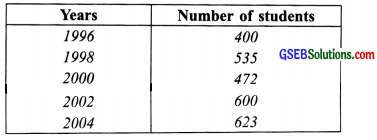A. Prepare a pictograph of students using one symbol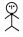to represent 100 students and answer the following questions:
(a) How many symbols represent the total number of students in the year 2002?
(b) How many symbols represent the total number of students for the year 1998?B. Prepare another pictograph of students using any other symbol each representing 50. students. Which pictograph do you find more informative?
Solution:
A.We have the following pictograph using= 100 students.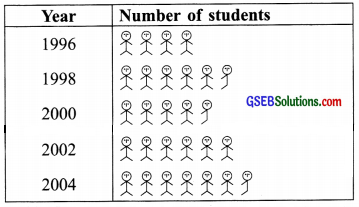(a) In the year 2002, a total number of students is represented by 6 symbols.
B. By taking 5 = 50, we prepare another pictograph as shown below: